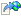﻿IWhereCondition(TParent) Members
 IWhereCondition TParent  Members Kentico 8.1 API Reference
The IWhereCondition TParent  type exposes the following members.
Methods

NameDescriptionAnd
Changes the where operator to AND for subsequent where conditions. Use in combination of methods Where...And(IWhereCondition)
Adds the given where condition with the AND operatorAnd(Action WhereCondition )
Adds the given where condition with the AND operator. Creates a new where condition object and runs the setup actions on it.ApplyParametersTo
Applies this where condition to the target object
(Inherited from IQueryParameters.)CloneObject
Creates the clone of the object.
(Inherited from IQueryObject.)Dispose
Performs application-defined tasks associated with freeing, releasing, or resetting unmanaged resources.
(Inherited fromIDisposable.)EnsureParameters
Ensures data parameters for the given query
(Inherited from IQueryParameters.)Expand
Expands the expression by replacing parameters with their values
(Inherited from IQueryParameters.)Changed
Marks the object as changed
(Inherited from IQueryObject.)IncludeDataParameters
Adds the data parameters to the current query parameters
(Inherited from IQueryObject.)NewWhere
Clears the current where conditionNoResults
Sets the where condition to exclude all data from resultOr
Changes the where operator to OR for next where conditions. Use in combination of methods Where...Or(IWhereCondition)
Adds the given where condition with the OR operatorOr(Action WhereCondition )
Adds the given where condition with the OR operator. Creates a new where condition object and runs the setup actions on it.ReturnNoResults
Sets the query to return no results. This action is irreversible, once the query is set to return no results it cannot be changed. This method is used by data engine to forbid access to data that are not allowed to be accessed (e.g. license limitations), without notifying the process about the fact.
(Inherited from IWhereCondition.)ToString
Returns the string representation of the expression, with possibility of expanding parameters
(Inherited from IQueryParameters.)Where( IWhereCondition )
Adds the given where conditions to the queryWhere(Action WhereCondition )
Adds the given where condition to the query. Creates a new where condition object and runs the setup actions on it.Where(String, QueryDataParameters)
Adds the given where condition to the queryWhere(String, QueryUnaryOperator)
Adds the given where condition to the query. Matches the column value with an unary operator.Where(String, QueryOperator, Object)
Adds the given where condition to the query. Matches the column to a given value.WhereContains
Adds the condition for a string column to contain some substringWhereEmpty
Adds the where condition for a null or empty column valueWhereEquals
Adds the given where condition to the query. Matches the column to a given value.WhereEqualsOrNull
Adds the given where condition to the query. Matches the column to a given value or null value.WhereExists
Adds where condition with EXISTS and the nested query "EXISTS (...)"WhereFalse
Adds the where condition for a false column value (boolean column equals false).WhereID
Adds the where condition to match the ID to the query. In case the column name is not provided or unknown, does not generate where condition. If given ID is invalid, adds the condition to match NULL.WhereIn(String, IDataQuery)
Adds where condition to the nested query, e.g. "columnName IN (...)"WhereIn(String, IList Int32 )
Adds where condition to the list of values, e.g. "columnName IN (...)"WhereLike
Adds the given where condition to the query. Matches the column to a given value using LIKE operator.WhereNot
Adds the negation of the given where conditionWhereNotContains
Adds the condition for a string column not to contain some substringWhereNotEmpty
Adds the where condition for a non empty column valueWhereNotExists
Adds where condition with NOT EXISTS and the nested query "NOT EXISTS (...)"WhereNotIn(String, IDataQuery)
Adds where condition to the nested query, e.g. "columnName NOT IN (...)"WhereNotIn(String, IList Int32 )
Adds where condition to the list of values, e.g. "columnName NOT IN (...)"WhereNotIn(String, IList String )
Adds where condition to the list of values, e.g. "columnName NOT IN (...)"WhereNotLike
Adds the given where condition to the query. Matches the column to a given value using NOT LIKE operator.WhereNotNull
Adds the where condition for a not null column valueWhereNotStartsWith
Adds the condition for a string column not to start with some prefixWhereNull
Adds the where condition for a null column valueWhereStartsWith
Adds the condition for a string column to start with some prefixWhereTrue
Adds the where condition for a true column value (boolean column equals true).
Back to Top
Properties

NameDescriptionName
Object name, empty by default
(Inherited from IQueryObject.)Parameters
Query data parameters
(Inherited from IQueryObject.)WhereCondition
Where condition on the data, e.g. "DocumentName = 'ABC'"
(Inherited from IWhereCondition.)WhereIsComplex
Returns true if the given where condition is a complex where condition
(Inherited from IWhereCondition.)
Back to Top
See Also